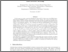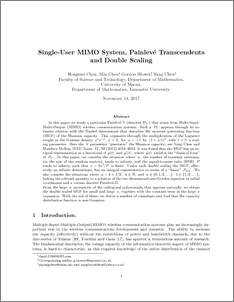# Single uer MIMO system, Painleve transcendents and double scaling

Chen, Hongmei and Chen, Min and Blower, Gordon and Chen, Yang (2017) Single uer MIMO system, Painleve transcendents and double scaling. Journal of Mathematical Physics, 58 (12). ISSN 0022-2488Preview
PDF (chenchenblowerchenJmathPhysrev)
chenchenblowerchenJmathPhysrev.pdf - Accepted Version

## Abstract

In this paper we study a particular Painlev\'e V (denoted ${\rm P_{V}}$) that arises from Multi-Input-Multi-Output (MIMO) wireless communication systems. Such a $P_V$ appears through its intimate relation with the Hankel determinant that describes the moment generating function (MGF) of the Shannon capacity. This originates through the multiplication of the Laguerre weight or the Gamma density $x^{\alpha} {\rm e}^{-x},\;x> 0,$ for $\alpha>-1$ by $(1+x/t)^{\lambda}$ with $t>0$ a scaling parameter. Here the $\lambda$ parameter generates" the Shannon capacity; see Yang Chen and Matthew McKay, IEEE Trans. IT, 58 (2012) 4594--4634. It was found that the MGF has an integral representation as a functional of $y(t)$ and $y'(t)$, where $y(t)$ satisfies the classical form" of $P_V$. In this paper, we consider the situation where $n,$ the number of transmit antennas, (or the size of the random matrix), tends to infinity, and the signal-to-noise ratio (SNR) $P$ tends to infinity, such that $s={4n^{2}}/{P}$ is finite. Under such double scaling the MGF, effectively an infinite determinant, has an integral representation in terms of a lesser" $P_{III}$. We also consider the situations where $\alpha=k+1/2,\;\;k\in \mathbb{N},$ and $\alpha\in\{0,1,2,\dots\}$ $\lambda\in\{1,2,\dots\},$ linking the relevant quantity to a solution of the two dimensional sine-Gordon equation in radial coordinates and a certain discrete Painlev\'e-II. \\ From the large $n$ asymptotic of the orthogonal polynomials, that appears naturally, we obtain the double scaled MGF for small and large $s$, together with the constant term in the large $s$ expansion. With the aid of these, we derive a number of cumulants and find that the capacity distribution function is non-Gaussian.

Item Type:
Journal Article
Journal or Publication Title:
Journal of Mathematical Physics
Copyright 2017 American Institute of Physics. The following article appeared in Journal of Mathematical Physics, 58, 12 2017 and may be found at http://dx.doi.org/10.1063/1.5017127 This article may be downloaded for personal use only. Any other use requires prior permission of the author and the American Institute of Physics.
Uncontrolled Keywords:
/dk/atira/pure/subjectarea/asjc/3100/3109
Subjects:
Departments:
ID Code:
88961
Deposited By:
Deposited On:
27 Nov 2017 09:22
Refereed?:
Yes
Published?:
Published